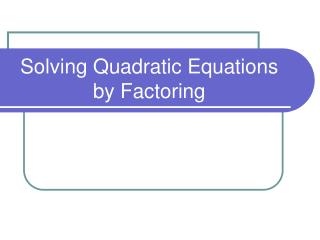DownloadDownload Presentation# Solving Quadratic Equations by Factoring

Download Presentation## Solving Quadratic Equations by Factoring

- - - - - - - - - - - - - - - - - - - - - - - - - - - E N D - - - - - - - - - - - - - - - - - - - - - - - - - - -
##### Presentation Transcript

1. Solving Quadratic Equations by Factoring

2. Solving Quadratic Equations by Factoring • The quadratic equation is written in the form ax2 + bx + c = 0 • To solve quadratic equations by factoring we use the Zero Product Principle If AB = 0 then A = 0 or B = 0  Where A and B are two quantities

3. Solving Quadratic Equations by Factoring Example: Solve each of the following quadratic equations. • x2 – 3x – 40 = 0 (x – 8)(x + 5) = 0 x – 8 = 0 or x + 5 = 0  Zero Product Principle x = 8 or x = -5 The solution set s.s. = {8, -5}

4. Solving Quadratic Equations by Factoring b) 8x2 = 15 – 14x 8x2 + 14x – 15 = 0 (4x – 3)(2x + 5) = 0 4x – 3 = 0 or 2x + 5 = 0

5. Solving Quadratic Equations by Factoring c) 3x2 – 9x = 0 3x(x – 3) = 0 3x = 0 or x – 3 = 0 x = 0 or x = 3 s.s. = {0, 3}

6. Homework • Do # 1 – 15 odd #’s only and # 19 and 21 on page 165 from section 5.5 for Tuesday May 12th